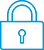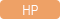cancel
Showing results for
Search instead for
Did you mean:It has been a while since anyone has replied. Simply ask a new question if you would like to start the discussion again.New member
4 3 0 0
Message 1 of 7
1,403
Flag Post

# HP Prime Speeadsheet Syntax ErrorsHP Recommended
HP Prime Calculator v2
Microsoft Windows 7 (64-bit)

I'm trying to compose a supposedly simple program into the Spreadsheet App on a new version HP Prime Calculator.  In column A are the numerical values. Column B has the cells named for the variables in Column A.  Column C has the outputs named and Column D has the equations that reference the variables in Column A.   It looks something like this:

A                B                C                 D

1   8.6          pH               pHs           equation 1

2   352        Calcium      LSI            equation 2

3   125        M-Alk          Ryzar      equation 3

4   1400      Cond.         Puckrs.    equation 4

5    140       Temp F

equation 1 is:

=9.4-LOG(A2)-0.94*LOG(A3)+LOG(A4)/10.7+3.24*LN(-(A5)/191)

equation 2 is:

=A1-D1

I keep getting a syntax error when I press "Enter" after writing the equation. What do I need to do differently?

Also, is there a way to set up the sheet on my laptop in the Connectvity Kit and save it to the calculator?  I can open a spreadsheet from the list of apps in the connectivity kit but I'm not seeing a way to save it.

Thanks!!

Tags (4)
6 REPLIES 6Level 8
622 609 117 207
Message 2 of 7
Flag PostHP Recommended

After entering your A1 through A5 data into the spreadsheet, I copied your equation 1 and pasted it directly as-is into the Virtual Prime, and it reported "Error: Invalid input" because the final LN is negative.  Removing the negative sign there yields a valid result (4.16994812421). At no time was there a "syntax error".

Equation 2 yields 4.43005187579 immediately. No error.

Not sure why you are getting a syntax error. Perhaps a typo? (An error would occur if your Prime were in RPN entry mode, of course, but I'll assume that's not the case because your equations are algebraic).

EDIT: In case your Prime is in RPN mode, you can still enter formulas into the spreadsheet by omitting the leading "=" sign and enclosing the formula in single quote marks.  Example: In place of

=A1-D1

type instead

'A1-D1'

-Joe-Level 6
305 297 32 76
Message 3 of 7
Flag PostHP Recommended

@sss-s wrote:

Also, is there a way to set up the sheet on my laptop in the Connectvity Kit and save it to the calculator?  I can open a spreadsheet from the list of apps in the connectivity kit but I'm not seeing a way to save it.

Thanks!!

Yes, you can save it by pressing the Save icon on the toolbar at the upper left side of the screen (third icon in my case).

I advise to reset the Spreadsheet first, before going to edit it.

The connectivity kit offers less editing and viewing  possibilities though than the calculator.New member
4 3 0 0
Message 4 of 7
Flag PostHP Recommended

Joe:

Thanks for your help, the calculator was in RPN, that's my preferred mode.  I entered in the equations in the single italics (Shift+() key) and the formulas entered but no values show - only the formula.  in fact, after I hit enter, the equation has an equal sign in front of it in addition to the single italics.  Also, there's CAS written in red capitals over the equal sign on the entry line (bottom of the screen) for the equations that are longer than the cell.

So I enter:

'9.4-LOG(A2)-0.94*LOG(A3)+LOG(A4)/10.7+3.24*EXP(-(A5)/191)'

hit enter and get:

='9.4-LOG(A2)-0.94*LOG(A3)+LOG(A4)/10.7+3.24*EXP(-(A5)/191)'

in the cell, but no values.

Thanks.

ScottNew member
4 3 0 0
Message 5 of 7
Flag PostHP Recommended

Joe:

One more thing, if I use algebraic input to program the HP Prime, instead of RPN, save it  and come back to it later with the calculator in RPN mode, will it run properly then?  Thanks.

ScottLevel 8
747 744 121 187
Message 6 of 7
Flag PostHP Recommended

You are trying to evaluate with the CAS. I suspect that is the problem here. Edit your formulas that have that "CAS" in there, click the CAS button on the menu to NOT evaluate with the CAS and it will probably work. I'm guessing you accidentally hit that somehow during an entry.

The reason it still shows as =<formula> even in RPN mode is that we are dealing with a spreadsheet. There is no concept of an RPN spreadsheet anywhere really, and ALL spreadsheets use that form to represent formulas.

Yes, it will work. All that is happening is that a formula is being stored for that cell. Either in RPN or alg the formulas are identical internally.

TW

Although I work for the HP calculator group as a head developer of the HP Prime, the views and opinions I post here are my own.New member
4 3 0 0
Message 7 of 7
Flag PostHP Recommended

Joe:

it it did the trick. Thank you very much.

Scott

† The opinions expressed above are the personal opinions of the authors, not of HP. By using this site, you accept the Terms of Use and Rules of Participation